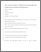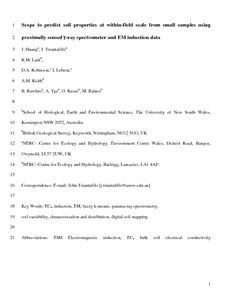nerc.ac.uk

Scope to predict soil properties at within-field scale from small samples using proximally sensed γ-ray spectrometer and EM induction data

Huang, J.; Lark, R.M.; Robinson, D.A.; Lebron, I.; Keith, A.M.; Rawlins, B.; Tye, A.; Kuras, O.; Raines, M.; Triantafilis, J.. 2014 Scope to predict soil properties at within-field scale from small samples using proximally sensed γ-ray spectrometer and EM induction data. Geoderma, 232-234. 69-80. https://doi.org/10.1016/j.geoderma.2014.04.031Preview
Text
Huang et al 2014.pdf - Accepted Version

Abstract/Summary

Spatial predictions of soil properties are needed for various purposes. However, the costs associated with soil sampling and laboratory analysis are substantial. One way to improve efficiencies is to combine measurement of soil properties with collection of cheaper-to-measure ancillary data. There are two possible approaches. The first is the formation of classes from ancillary data. A second is the use of a simple predictive linear model of the target soil property on the ancillary variables. Here, results are presented and compared where proximally sensed gamma-ray (γ-ray) spectrometry and electromagnetic induction (EMI) data are used to predict the variation in topsoil properties (e.g. clay content and pH). In the first instance, the proximal data is numerically clustered using a fuzzy k-means (FKM) clustering algorithm, to identify contiguous classes. The resultant digital soil maps (i.e. k = 2–10 classes) are consistent with a soil series map generated using traditional soil profile description, classification and mapping methods at a highly variable site near the township of Shelford, Nottinghamshire UK. In terms of prediction, the calculated expected value of mean squared prediction error (i.e. σ2p,C) indicated that values of k = 7 and 8 were ideal for predicting clay and pH. Secondly, a linear mixed model (LMM) is fitted in which the proximal data are fixed effects but the residuals are treated as a combination of a spatially correlated random effect and an independent and identically distributed error. In terms of prediction, the expected value of the mean squared prediction error from a regression (σ2p,R) suggested that the regression models were able to predict clay content, better than FKM clustering. The reverse was true with respect to pH, however. We conclude that both methods have merit. In the case of the clustering the approach is able to account for soil properties which have non-linearity's with the ancillary data (i.e. pH), whereas the LMM approach is best when there is a strong linear relationship (i.e. clay).

Item Type: Publication - Article https://doi.org/10.1016/j.geoderma.2014.04.031 Emmett 00167061 26 Aug 2014 13:44 +0 (UTC) http://nora.nerc.ac.uk/id/eprint/508192View Item